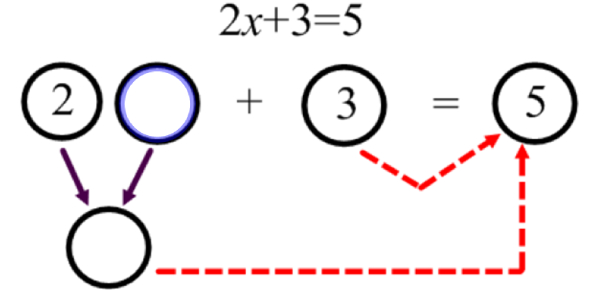# Algebra Trivia: MCQ Quiz! Exam

Approved & Edited by ProProfs Editorial Team
At ProProfs Quizzes, our dedicated in-house team of experts takes pride in their work. With a sharp eye for detail, they meticulously review each quiz. This ensures that every quiz, taken by over 100 million users, meets our standards of accuracy, clarity, and engagement.
| Written by Bcankar
B
Bcankar
Community Contributor
Quizzes Created: 1 | Total Attempts: 79
Questions: 8 | Attempts: 79Settings.

• 1.

• A.
• B.
• C.
• D.
A.
• 2.

### Which of the following is the standard form equation of a circle with center (2, 3) that passes through the point  (4, -2)?

• A.
• B.
• C.
• D.
A.
Explanation
The standard form equation of a circle is (x - h)^2 + (y - k)^2 = r^2, where (h, k) represents the center of the circle and r represents the radius. In this case, the center of the circle is (2, 3), so the equation starts as (x - 2)^2 + (y - 3)^2 = r^2. Since the circle passes through the point (4, -2), we can substitute these values into the equation to find the radius. By substituting (4 - 2)^2 + (-2 - 3)^2 = r^2, we get (2)^2 + (-5)^2 = r^2. Simplifying this gives us 4 + 25 = r^2, or 29 = r^2. Therefore, the standard form equation of the circle is (x - 2)^2 + (y - 3)^2 = 29.

Rate this question:

• 3.

### What distance does the minute hand of a clock travel in 45 minutes if its length is 12 inches?

• A.
• B.
• C.
• D.
A.
Explanation
The minute hand of a clock travels in a circular motion around the clock face. The circumference of a circle is calculated by multiplying the diameter by π (pi). In this case, the length of the minute hand is given as 12 inches, which is equal to the diameter of the circle it travels. Therefore, the distance traveled by the minute hand in 45 minutes is equal to the circumference of the circle, which is 12π inches.

Rate this question:

• 4.

• A.
• B.
• C.
• D.
A.
• 5.

### The solution to the equation  is x = ____________

4
Explanation
The solution to the equation is x = 4 because when the equation is solved, the variable x is equal to 4.

Rate this question:

• 6.

### How many increasing intervals does the following graph have?

• A.

3

• B.

2

• C.

1

• D.

0

A. 3
Explanation
The graph has three increasing intervals because there are three distinct sections where the values are increasing. Each time the graph transitions from a lower value to a higher value, it represents a new increasing interval.

Rate this question:

• 7.

### Describe the boundedness for the graph below:

• A.

Not bounded

• B.

Bounded above

• C.

Bounded below

• D.

Bounded

A. Not bounded
Explanation
The graph is not bounded because it extends indefinitely in at least one direction. It does not have a specific upper or lower limit, meaning it can continue to increase or decrease without bound. Therefore, it is not bounded above or below, and overall, it is not bounded.

Rate this question:

• 8.

### What is the exact value of ?

• A.
• B.
• C.
• D.Back to top